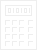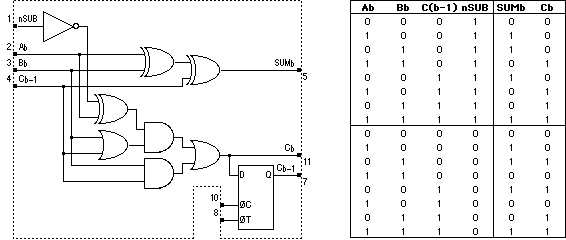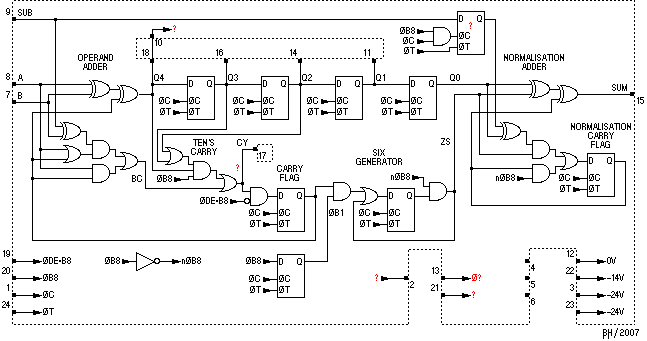EarlyElectronicCalculator JMOS Serial AdderIntegrated CircuitsThe JMOS family of IC's includes some serial adder MSI devices. Following are the presumed or functionally equivalent internal structure of these devices.

### TM4006

The TM4006 is a serial adder including a carry latch. This is standard construction for a serial adder except for the addition of the subtraction facility. When pin 1 is FALSE the B operand is subtracted from the A operand.

See the Toshiba BC-1212 for an example of it's use.

 TM4006 Serial AdderFunctional Equivalent of Internal Structure### HD3112

The HD3112 is considerably more complex, providing BCD sum correction as well as subtraction. As shown below, it is presumed to contain two serial adders, a 4-bit shift register and additional logic for subtraction, ten's carry detection and the BCD sum correction.

The first adder adds or subtracts the two input operands. For subtraction, A is subtracted from B (B-A). The raw sum for a digit is collected in the 4-bit shift register. At the end of the digit, as determined by the ØB8 input, the shift register state along with the bit carry is used to make the carry out of the digit into a ten's carry. The second adder performs the BCD sum correction (normalisation) by adding or subtracting 6 to the digit coming out of the shift register if a digit carry was detected.

Notice that the carry circuitry for the normalisation adder is simplified from that of the operand adder. This is possible because one input is limited to being either 0 or 6 and the carry into the 1 bit must be 0. Consequently:

• There can be no carry from the 1 bit into the 2 bit.
• If the carry flag is set to 1, it shall remain set for the remainder of the digit.
• The carry out of the 8 bit will not be used.
This optimisation was observed in the Sharp Compet 17 calculator.

The ØDE*B8 signal (DE=End Digit) is used to suppress the carry at the end of a number cycle from being fed in at the beginning of the next number cycle.

See the Commodore DAC-612 or Casio 121-B for examples of use of the HD3112.

 HD3112 Serial BCD AdderFunctional Equivalent of Internal StructureSeveral aspects of this device remain undeciphered:

• Pin 17 is presumed to be a Carry output but at exactly which point in the carry path it takes off from has not been determined.
• Pin 10 is connected to pin 18 in both calculators. It may be that one pin is the output from the operand adder and the other is the input to the shift register.
• The flip-flop in the subtract line may or may not be present. ItŐs objective is to delay the SUB signal to appropriately affect or not affect the BCD digit already in the shift register.
• Pins 2, 13 and 21 are used differently in the Commodore versus the Casio. Their functions have not been determined.
• Pin 2 does not seem to show any activity in the Commodore.
• Pin 13 receives the ØC clock, gated with another signal in the Commodore.
• Guess: Pin 21 enables/disables the influence of the ten's carry into the carry flag.
• Pins 4, 5 and 6 are not connected in both calculators.

 JMOS Serial Adders Calculators | Integrated Circuits | Displays | Simulations EEC 2007 Jan